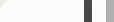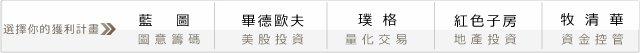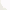## 第一次程式交易就上手－如何建構第一支自己的程式#### 參數與變數設定

input : R(0.002),BeginTime(0915),EndTime(1245);

Input後面是接我們程式裡可變動的參數，

R是代表區間上下的某個比例，
BeginTime是我們開始交易的時間，
EndTime是我們終止交易的時間。

var: TH(0),TL(0),mkp(0),ax(0),ay(0);

TH是用來儲存我們當日某段時間裡的最高點；
TL是用來儲存我們當日某段時間裡的最低點。
mkp用來儲存我們手邊部位狀態。
ax用來計算我們作多的次數，
ay用來計算我們作空的次數。
ax跟ay可以用來限制我們當日多空交易次數。

if date <> date then begin
mkp=0;
ax=0;
ay=0;
end;

#### 進場方式if BeginTime < Time and Time < EndTime then begin
if MarketPosition = 0 and high > TH*(1+R) then buy next bar at highest(high,1)+1 stop;
if MarketPosition = 0 and low < TL*(1-R) then sell next bar at lowest(low,1)-1 stop;
end;

mkp=marketposition;

if mkp<>1 and mkp=1 then ax=ax+1;

if mkp<>-1 and mkp=-1 then ay=ay+1;

ax就加1，表示作多一次，空單亦然。

#### 出場方式

if marketposition =1 then begin
exitlong at entryprice-50 stop ;
if time>1339 then exitlong this bar on close;
end;
if marketposition =-1 then begin
exitshort at entryprice+50 stop ;
if time>1339 then exitshort this bar on close;
end;
setexitonclose;

input : R(0.002),BeginTime(0915),EndTime(1245);

var :TH(0),TL(0),mkp(0),ax(0),ay(0);

if date <> date then begin
mkp=0;
ax=0;
ay=0;
end;
if time=BeginTime then begin
TH=highd(0);
TL=lowd(0);
end;

if BeginTime < Time and Time < EndTime then begin
if MarketPosition = 0 and ax < 2 and high > TH*(1+R) then buy at highest(h,1)+1 stop;
if MarketPosition = 0 and ay < 2 and low < TL*(1-R) then sell at lowest(l,1)-1 stop;
end;

mkp=marketposition;

if mkp <> 1 and mkp=1 then ax=ax+1;

if mkp <> -1 and mkp=-1 then ay=ay+1;

if marketposition =1 then begin
exitlong at entryprice-50 stop ;
if time>1339 then exitlong this bar on close;
end;

if marketposition =-1 then begin
exitshort at entryprice+50 stop ;
if time>1339 then exitshort this bar on close;
end;

setexitonclose;

#### 0 意見: